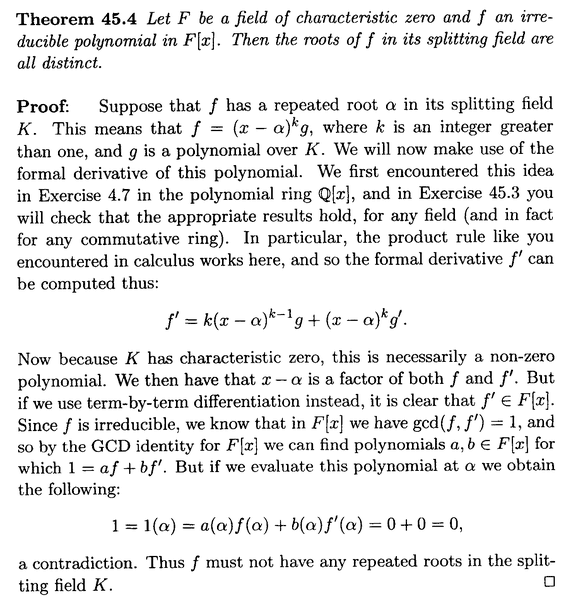# Splitting Fields: Anderson and Feil, Theorem 45.4 ...

Gold Member
I am reading Anderson and Feil - A First Course in Abstract Algebra.

I am currently focused on Ch. 45: The Splitting Field ... ...

I need some help with some aspects of the proof of Theorem 45.4 ...

Theorem 45.4 and its proof read as follows:My questions on the above proof are as follows:

Question 1

In the above text from Anderson and Feil we read the following:

"... ... This means that ##f = ( x - \alpha)^k g##, where ##k## is an integer greater than ##1## and ##g## is a polynomial over ##K## ... ...

Since ##f## is in ##F[x]## ... that is ##f## is over ##F## ... shouldn't ##g## be over ##F## not ##K##?

(I am assuming that ##f## being "over ##F##" means the coefficients of ##f## are in ##F## ... )

Question 2

In the above text from Anderson and Feil we read the following:

"... ... We then have that ##x - \alpha## is a factor of both ##f## and ##f'##. But if we use term-by-term differentiation instead, it is clear that ##f'\in F[x]##. ... ... "

What do Anderson and Feil mean by term-by-term differentiation in this context ... ... and if they do use term-by-term differentiation (what ever they mean) how does this show that ##f'\in F[x]## ... ... ?

Hope someone can help ...

Help will be much appreciated ... ...

Peter

#### Attachments

andrewkirk
Homework Helper
Gold Member
If ##\alpha\in K-F## then ##g## will not necessarily be in ##F## as its coefficients will be functions of ##\alpha##.

Consider ##F=\mathbb R,\ K=\mathbb C## and ##f(x)=(x^2+1)^2=(x-i)^2(x+i)^2##, which has roots ##i## and ##-i##, both of multiplicity 2. Taking ##\alpha=i## we get ##g(x)=(x+i)^2##, which is not in ##\mathbb R[x]##.

•Math Amateur
andrewkirk
Homework Helper
Gold Member
"... ... We then have that ##x - \alpha## is a factor of both ##f## and ##f'##. But if we use term-by-term differentiation instead, it is clear that ##f'\in F[x]##. ... ... "
I was going off to have dinner, thinking I didn't have time to work out the second one. Then I realised it's actually the easier of the two.

By 'term-by-term' they mean writing the polynomial in expanded, unfactorised form as ##f(x)=\sum_{k=0}^n c_kx^k## and then differentiating to get ##f'(x)=\sum_{k=1}^n kc_kx^{k-1}##. The coefficients are of the form ##kc_k## which, since ##k## is in ##\mathbb N## rather than ##F##, means ##\sum_{j=1}^k c_k##, which must be in ##F## since ##c_k## is. Hence ##f'\in F[x]##.

•Math Amateur and Greg Bernhardt
Gold Member
If ##\alpha\in K-F## then ##g## will not necessarily be in ##F## as its coefficients will be functions of ##\alpha##.

Consider ##F=\mathbb R,\ K=\mathbb C## and ##f(x)=(x^2+1)^2=(x-i)^2(x+i)^2##, which has roots ##i## and ##-i##, both of multiplicity 2. Taking ##\alpha=i## we get ##g(x)=(x+i)^2##, which is not in ##\mathbb R[x]##.

Thanks Andrew ... good example ... makes it pretty clear ...

Peter

•Greg Bernhardt